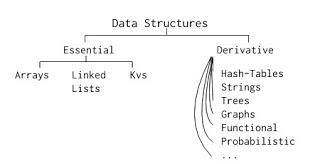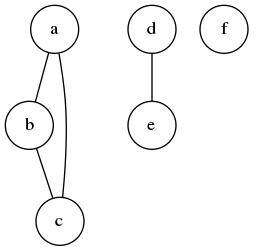# 通过 Lisp 语言理解编程算法：数据结构篇## 数据结构与算法

Eric S. Raymond 在《UNIX 编程艺术》（Art of Unix Programming）一书中，对同一个想法提出了不那么尖锐的版本，称之为“表示原则”（Rule of Representation）：

## 相连数据结构与链接数据结构

• 相连（contiguous）结构占用单个内存块，其内容存储在相邻的内存块中。要访问特定的块，我们应该知道它从分配给该结构的内存范围开始的偏移量。（这通常由编译器处理）。当处理器需要读写这一块时，它将使用指针，该指针作为结构的基址和偏移量之和来计算。相连结构的例子是数组和结构。
• 相反，链接结构不占用相连的内存块，即其内容位于不同的位置。这意味着只想特定块的指针不能预先计算，应该存储在结构本身中。这种结构更加灵活，但在访问某个元素所用的空间和时间方面都会增加额外的开销（当有嵌套时，可能需要多个跃点，而在相连结构中，它始终是常量）。存在大量链接数据结构，如列表、树和图。

## 元组

Python 有一个专用的元组数据类型，通常就是为了这个目的，它本质上是一个链接数据结构。下面的 Python 函数将返回一个十进制的元组（用括号编写）和数字 x 的其余部分：

def truncate(x):    dec = int(x)    rem = x - dec    return (dec, rem)

(defstruct pair  left right)

CL-USER> (make-pair :left "foo" :right "bar")#S(PAIR :LEFT "foo" :RIGHT "bar")CL-USER> (pair-right (read-from-string (prin1-to-string *)))"bar"

prin1-to-stringread-from-string 是互补的 Lisp 函数，它们允许以计算机可读的形式打印值（如果提供了适当的 print-function（打印函数）），并将其读取回来。理想情况下，良好的 print-representations 对人类和计算机来说都是可读的，对于代码透明度非常重要，不应该被忽视。

(defstruct (pair (:type list) (:conc-name nil))  "A generic pair with left (LT) and right (RT) elements."  lt rt) (defun pair (x y)  "A shortcut to make a pair of X and Y."  (make-pair :lt x :rt y))

## 作用中的结构：并查集(defstruct point  parent)  ; if the parent is null the point is the root (defun uf-union (point1 point2)  "Join the subsets of POINT1 and POINT2."  (:= (point-parent point1) (or (point-parent point2)                                point2))) (defun uf-find (point)  "Determine the id of the subset that a POINT belongs to."  (let ((parent (point-parent point)))    (if parent        (uf-find parent)        point)))

  d^ ^ ^| | |a b c

(defstruct point  parent  (size 1)) (defun uf-find (point)  (let ((parent (point-parent point)))    (if parent        ;; here, we use the fact that the assignment will also return        ;; the value to perform both path compression and find        (:= (point-parent point) (uf-find parent))        point))) (defun uf-union (point1 point2)  (with ((root1 (uf-find point1))         (root2 (uf-find point2))         (major minor (if (> (point-size root1)                             (point-size root2))                          (values root1 root2)                          (values root2 root1))))    (:+ (point-size major) (point-size minor))    (:= (point-parent minor) major)))

(defun uf-disjoint (points)  "Return true if all of the POINTS belong to different subsets."  (let (roots)    (dolist (point points)      (let ((root (uf-find point)))        (when (member root roots)          (return-from uf-disjoint nil))        (push root roots))))  t)

1. 我们最初的想法并不总是完美无缺的。检查边缘情况是否存在潜在问题是很重要的。
2. 我们已经目睹了一个压根就不存在的数据结构示例：信息片段分布在各个数据点上。有时，可以选择以集中的方式将信息存储在专用结构（如哈希表之类）中，或者将其分布存储在各个节点上。后一种方法通常更优雅，也更有效，尽管它并不是那么明显。

 此外，Python 有专门的语法来析构这类元组：dec, rem = truncate(3.14)。但是，这并不是处理从函数返回一次值和一个或多个二次值的最佳方法。所有必需的值都是通过 value 表单返回：(values dec rem) ，可以用 (multiple-value-bind (dec rem) (truncate 3.14) ...)(with ((dec rem (truncate 3.14))) ...) 来检索。它更优雅，因为可以通过通常方式调用函数来随意丢弃二次值：(+ 1 (truncate 3.14)) => 4：在 Python 中是不可能的，因为你不能用某个东西对元组进行求和。

 实际上，这里的复杂度是 O(n^2)，这是由于使用了在 O(n) 中执行集合成员测试的函数 member，但这对整个概念来说并不重要。如果期望使用数十个或数百个点调用 uf-disjoint，那么跟结构必须更改为具有 O(1) 成员操作的哈希集。

Vsevolod Dyomkin，Lisp 程序员，居住在乌克兰基辅。精通乌克兰语、俄语和英语。目前正在撰写关于 Lisp 的书籍 Programming Algorithms in Lisp，该书将使用 CC BY-NC-ND 许可（创作公用许可协议），供公众免费阅读。

LISP, THE UNIVERSE AND EVERYTHING